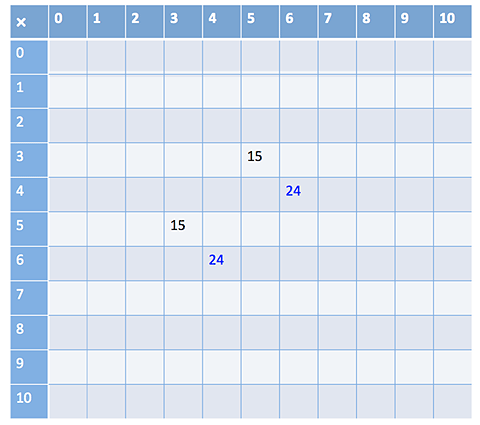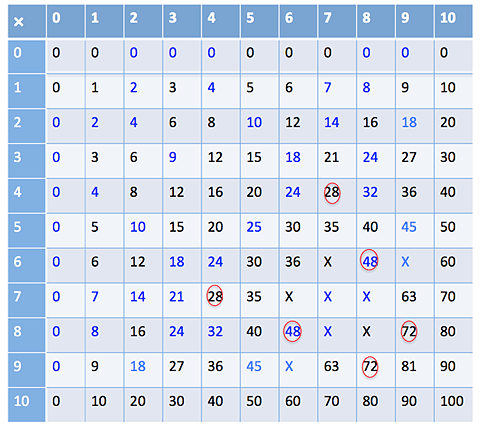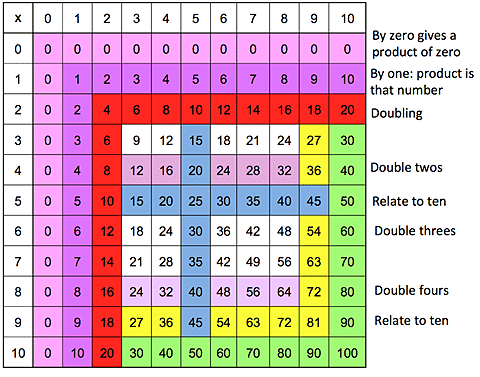# What do I know?

Developing an understanding of the properties of multiplication (such as the properties of 0, 1, commutativity) reduce the number of facts students need to remember.

### What do I know?

Materials: Blank multiplication grid and two 10-sided (0-9) dice per pair, coloured pencils.

• In pairs, students take turns to roll the dice and generate a multiplication fact.
For example, player A rolls (5, 3) and player B says "5 threes are 15, and 3 fives are 15".
• Player B records 15 in the appropriate cells on the grid, using a coloured pencil.Sample multiplication fact grid.

• If a player takes a long time to work out the product, it is recorded in the cell with a circle around it. If a player does not know then a cross is put in the cell.Completed multiplication fact grid.

• Play continues until all the cells are filled. Students should record the facts for 10 as they cannot be generated using the dice.
• Students discuss and list some of the strategies used for the facts that were tricky to work out.

You can watch the video What Do I Know?

You can download the What Do I Know? video transcript.

List some of the strategies students used for the facts that were tricky to work out (e.g. using 4 $$\times$$ 4 and doubles to work out 8 $$\times$$ 4).

List the facts that were not known. Discuss and list some strategies that might help learn these facts (e.g. 7 $$\times$$ 4 = 5 $$\times$$ 4 + 2 $$\times$$ 4).

Which facts are known without having to think? Shade these on a blank multiplication grid.

Which facts can be worked out using doubling or thinking of tens facts? Shade these on the grid.Shaded multiplication fact grid.

Those cells left uncoloured might include some square numbers and some threes, six and seven facts. Discuss the strategies the students might use to assist them to remember these facts.

You can download the What do I know? game rules as a slide presentation.